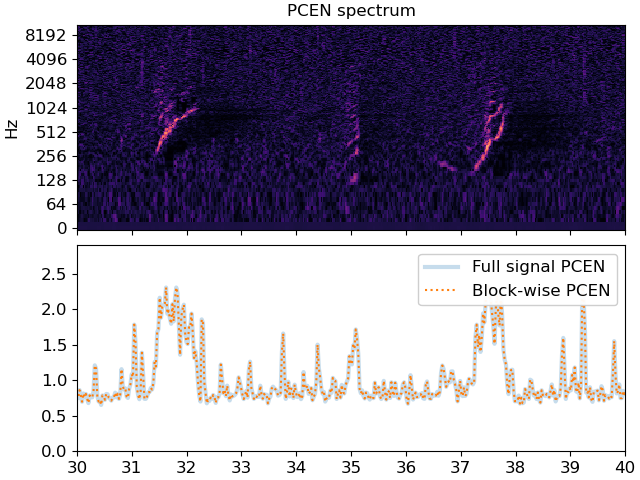# PCEN Streaming

This notebook demonstrates how to use streaming IO with `librosa.pcen` to do dynamic per-channel energy normalization on a spectrogram incrementally.

This is useful when processing long audio files that are too large to load all at once, or when streaming data from a recording device.

It also illustrates how to use a pre-allocated output buffer for block-wise short-time Fourier transforms. This provides a minor speed boost and reduction in memory usage when processing audio streams.

We’ll need numpy and matplotlib for this example

```import numpy as np
import matplotlib.pyplot as plt

import soundfile as sf

import librosa
```

First, we’ll start with an audio file that we want to stream

```filename = librosa.ex('humpback')
```

Next, we’ll set up the block reader to work on short segments of audio at a time.

```# We'll generate 64 frames at a time, each frame having 2048 samples
# and 75% overlap.
#

n_fft = 2048
hop_length = 512

# fill_value pads out the last frame with zeros so that we have a
# full frame at the end of the signal, even if the signal doesn't
# divide evenly into full frames.
sr = librosa.get_samplerate(filename)

stream = librosa.stream(filename, block_length=16,
frame_length=n_fft,
hop_length=hop_length,
mono=True,
fill_value=0)
```

For this example, we’ll compute PCEN on each block, find the maximum response over frequency, and store the results in a list.

```# Make an array to store the frequency-averaged PCEN values
pcen_blocks = []

# Initialize the PCEN filter delays to steady state
zi = None

# Create a handle for storing the block STFT outputs
# After the first block has been processed, we can re-use
# this buffer instead of allocating a new one for each block.
D = None

for y_block in stream:
# Compute the STFT (without padding, so center=False)
D = librosa.stft(y_block, n_fft=n_fft, hop_length=hop_length,
center=False, out=D)

# Compute PCEN on the magnitude spectrum, using initial delays
# returned from our previous call (if any)
# store the final delays for use as zi in the next iteration
P, zi = librosa.pcen(np.abs(D), sr=sr, hop_length=hop_length,
zi=zi, return_zf=True)

# Compute the max PCEN over frequency, and append it to our list
pcen_blocks.extend(np.max(P, axis=0))

# Cast to a numpy array for use downstream
pcen_blocks = np.asarray(pcen_blocks)
```

For the sake of comparison, let’s see how it would look had we run PCEN on the entire spectrum without block-wise processing

```y, sr = librosa.load(filename, sr=sr)

# Keep the same parameters as before
D = librosa.stft(y, n_fft=n_fft, hop_length=hop_length, center=False)

# Compute pcen on the magnitude spectrum.
# We don't need to worry about initial and final filter delays if
# we're doing everything in one go.
P = librosa.pcen(np.abs(D), sr=sr, hop_length=hop_length)

pcen_full = np.max(P, axis=0)
```

Plot the PCEN spectrum and the resulting magnitudes

```# First, plot the spectrum
fig, ax = plt.subplots(nrows=2, sharex=True)
librosa.display.specshow(P, sr=sr, hop_length=hop_length,
x_axis='time', y_axis='log', ax=ax)
ax.set(title='PCEN spectrum')
ax.label_outer()

# Now we'll plot the pcen curves
times = librosa.times_like(pcen_full, sr=sr, hop_length=hop_length)
ax.plot(times, pcen_full, linewidth=3, alpha=0.25, label='Full signal PCEN')
times = librosa.times_like(pcen_blocks, sr=sr, hop_length=hop_length)
ax.plot(times, pcen_blocks, linestyle=':', label='Block-wise PCEN')
ax.legend()

# Zoom in to a short patch to see the fine details
ax.set(xlim=[30, 40]);
```Total running time of the script: (0 minutes 3.436 seconds)

Gallery generated by Sphinx-Gallery